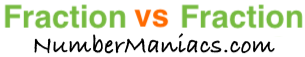4/5 vs 7/8People compare 4/5 vs 7/8 drywall, 4/5 vs 7/8 pipe, 4/5 vs 7/8 cup, 4/5 vs 7/8 ton, 4/5 vs 7/8 drill bit, 4/5 vs 7/8 poker, 4/5 vs 7/8 sump pump, 4/5 vs 7/8 garden hose, and many other items.

Is 4/5 greater than 7/8? Is 4/5 less than 7/8? What is the difference between 4/5 and 7/8? These and other questions are answered below so you can get a better perspective of 4/5 vs 7/8.

4/5 vs 7/8 Fractional Comparisons
4/5 is less than 7/8
The difference between 4/5 and 7/8 is 3/40
4/5 is 3/40 less than 7/8

4/5 vs 7/8 Decimal Comparisons
4/5 vs 7/8 as a decimal is 0.8 vs 0.875
The difference between 4/5 and 7/8 is 0.075
4/5 is 0.075 less than 7/8

4/5 vs 7/8 Percent Comparisons
4/5 vs 7/8 as a percent is 80% vs 87.5%
4/5 is 8.571 percent less than 7/8

Fraction vs Fraction
Submit another set of fractions that we can compare for you!

/
vs
/

The math behind the numbers
Calculations on this page are rounded to the nearest thousandth if necessary. Below is the math we used to analyze 4/5 vs 7/8:

To compare the size of 4/5 vs 7/8, we simply subtracted 7/8 from 4/5:

4/5 - 7/8 = |3/40|

To find 4/5 vs 7/8 as a decimal, we divided the numerator by the denominator for each fraction.

4 ÷ 5 = 0.8
7 ÷ 8 = 0.875

To find 4/5 vs 7/8 as a percent, we divided the numerator by the denominator and then multiplied the quotient by 100 for each fraction.

(4 ÷ 5) x 100 = 80%
(7 ÷ 8) x 100 = 87.5%

We found that 4/5 is 8.571 percent less than 7/8 by dividing the difference between the two fractions by the second fraction and then multiplied it by 100:

((3/40)/(7/8))×100 = 8.571%

That's it folks. We hope this page gave you a better understanding of 4/5 vs 7/8 with our explanations and conclusions.

4/5 vs 7/9
Here are the next two fractions we have compared and analyzed.